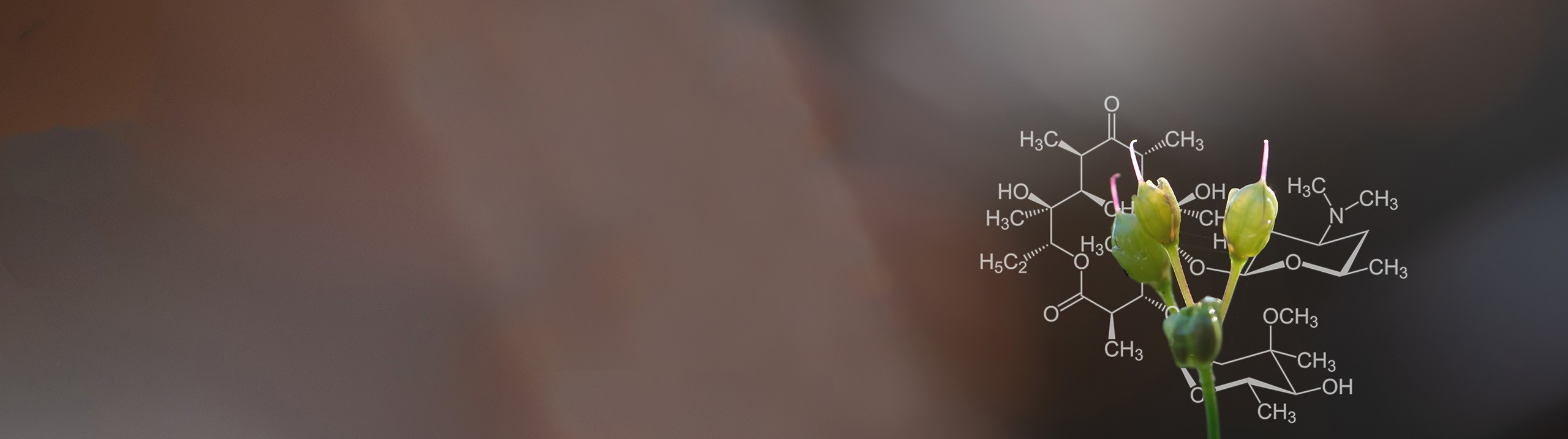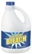Start typing, then use the up and down arrows to select an option from the list.# Biochemistry

Learn the toughest concepts covered in Biochemistry with step-by-step video tutorials and practice problems by world-class tutors

4. Protein Structure

# Determining Net Charge of a Peptide

1

#### concept

Determining Net Charge of a Peptide8m
Play a video:
2
Problem

What is the net charge if you drop the peptide above into bleach (pH 12)?3
Problem

Answer the following questions (A, B & C) relating to the 4 tripeptides.

i) Tyr-Lys-Met                    ii) Asp-Trp-Tyr                    iii) Asp-His-Glu                  iv) Leu-Val-Phe

A. Which tripeptide is most negatively charged at pH = 7? _________

B. Which tripeptide contains the largest number of nonpolar R groups? __________

C. Which tripeptide contains sulfur? ____________

4
Problem

Estimate the net charge for a His-His-His-His peptide at pH 6 (His pK  R = 6).

5
Problem

Estimate the net charge for the following peptide at pH 7: ATLDAK.

6
Problem

A. Draw the predominant structure of the following peptide at pH 9: Asn-Arg-Cys. What is its net charge?

(Asn pKa1 = 8.8, Arg pKR = 12.48, Cys pKa2 = 1.96, Cys pKR = 8.18).

B. What is the net charge of the same peptide if the pH is lowered to pH = 2? Draw the newly ionized peptide.

C. Within what pH range would the net charge on the peptide above be approximately +1?

pH: ______________